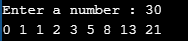# For Loop in C Language

## For Loop in C Language with Examples

In this article, I will discuss the For loop in C Language with Examples. Please read our previous articles discussing the Do While loop in C Language with Examples. The for loop is one of the most commonly used loops in the C# language. At the end of this article, you will understand what a for loop is and when and how to use a for loop in the C program with examples.

##### For Loop in C Language:

The for loop in C is a control structure that allows code to be executed repeatedly based on a condition. It’s typically used for iterating over arrays or performing a task several times. The for loop is particularly useful for cases where the number of iterations is known beforehand. That means if we know the number of times we want to execute some statements or instructions, we should use a for loop. The for loop is known as a Counter loop. We need to use a for loop whenever counting is involved for repetition.

##### For Loop Flowchart

The following diagram shows the flowchart of the for loop.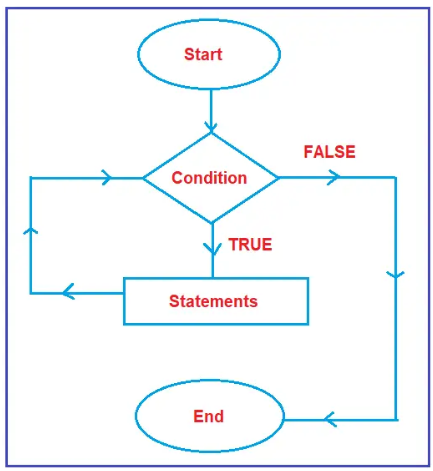The flow chart will start. The oval symbol represents the start. Then, it will check the condition. As discussed earlier, every condition has two outputs, i.e., true and false. What will happen if it is true, and what will happen if it is false? We need to check.

Suppose the condition is true, then all statements defined inside the block (within the for-loop block) will execute. After the execution of statements, will it end? No, it will not end. After executing statements, it will once again check the for-loop condition. It will repeat the same process if the given loop condition is true. And when the condition becomes false, then it will come to an end. This is the execution flow of the for loop in C#.

##### Syntax to use for loop in C Language:

A for loop in C is a control flow statement that allows code to be executed repeatedly based on a given Boolean condition. The syntax of a for loop in C is as follows:

```for (initialization; condition; increment/decrement) {
// code to be executed
}
```

Here,

• Initialization: It is executed before the loop starts. It is often used to initialize a counter variable.
• Condition: This is the Boolean condition checked before every loop iteration. If the condition is true, the loop continues; if false, the loop stops.
• Increment/Decrement: This statement is executed after each loop iteration. It is commonly used to update the counter variable.
###### Here’s an example of a for loop in C:
```#include <stdio.h>

int main() {
int i;
for (i = 0; i < 10; i++) {
printf("%d\n", i);
}
return 0;
}
```

In this example, the for loop initializes i to 0, running as long as i is less than 10. After each iteration, i is incremented by 1. The loop prints the numbers from 0 to 9.

##### For Loop Execution Flow in C

The execution flow of a for loop in C can be understood through the following steps:

• Initialization Step: Before the first iteration of the loop, the initialization statement is executed. This is typically used to initialize a counter variable. This step is executed only once.
• Condition Check: After the initialization step, the condition is evaluated. This is a Boolean expression that determines whether the loop should execute. If the condition is true, the loop executes the statements inside its body. If the condition evaluates to false, the loop terminates, and the control moves to the statement following the loop.
• Execution of Loop Body: If the condition is true, the statements inside the loop body are executed.
• Increment/Update Step: The update statement (often an increment or decrement operation) is executed after the loop body is executed. This step is crucial for changing the state of the loop control variable, leading to a re-evaluation of the condition.
• Condition Re-check: The condition is checked again. If it is still true, steps 3 and 4 are repeated. If it is false, the loop terminates.
• Exit the Loop: Once the condition becomes false, the loop stops and the program continues with the next statement after the loop.
##### Example: For Loop with Multiple Initialization and Update Expressions

A for loop can have multiple initialization and update expressions, separated by commas.

```#include <stdio.h>

int main() {
int i, j;
for (i = 0, j = 10; i <= 10 && j >= 0; i++, j--) {
printf("i = %d, j = %d\n", i, j);
}
return 0;
}```
##### Example: For Loop for Array Iteration

This example uses a for loop to iterate over an array.

```#include <stdio.h>

int main() {
int arr[] = {10, 20, 30, 40, 50};
int i;
for (i = 0; i < 5; i++) {
printf("%d\n", arr[i]);
}
return 0;
}```
##### Example: Infinite For Loop

An infinite loop runs forever unless interrupted. This is achieved by omitting the condition.

```#include <stdio.h>

int main() {
for (;;) {
printf("This loop will run forever.\n");
}
return 0;
}```
##### Example: For Loop with Break Statement

Using break to exit a for loop prematurely.

```#include <stdio.h>

int main() {
int i;
for (i = 1; i <= 10; i++) {
if (i == 6) {
break;
}
printf("%d\n", i);
}
return 0;
}```
##### Example: For Loop with Continue Statement

Using continue to skip the current iteration and proceed to the next.

```Using continue to skip the current iteration and proceed to the next.

#include <stdio.h>

int main() {
int i;
for (i = 1; i <= 10; i++) {
if (i % 2 == 0) {
continue;
}
printf("%d\n", i);
}
return 0;
}```
##### Real-Time Example: Perfect Number

Let us create a Program to Enter a Number and Check Whether that Number is a Perfect Number or Not. If the sum of all factors equals that number, it is called a perfect number.

```#include <stdio.h>
int main()
{
int n, i, sum = 0;
printf("\nenter a number ");
scanf("%d", &n);
for(i = 1; i<= n/2; i++)
{
if(n % i == 0)
sum = sum + i;
}
if (sum == n && n != 0)
printf("it is a perfect number");
else
printf("it is not a perfect number");
return 0;
}```
###### Output: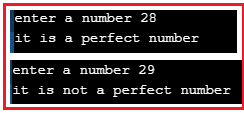##### Example: Armstrong Number

Let us Create a Program to check whether a number is Armstrong no or not using C Language. If the sum of all individual cube values equals that number, it is called the Armstrong number.

```#include <stdio.h>
int main()
{
int n, rem, temp, sum = 0;
printf("\nEnter a number : ");
scanf("%d", &n);
for(temp = n; temp != 0;)
{
rem = temp % 10;
sum = sum + (rem* rem* rem);
temp = temp /10;
}
if (sum == n && n != 0)
printf("It is an Armstrong number");
else
printf("It is not an Armstrong number");
return 0;
}
```
###### Output: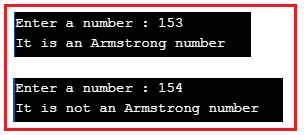##### Example: Prime Number

Let us create a Program to enter a number and check whether it is a prime number or not using a for loop in C Language. The number divisible by 1 and itself is called a prime number.

```#include <stdio.h>
int main()
{
int n, i;
printf("\nEnter a number : ");
scanf("%d", &n);
for(i = 2; i < n; i++)
{
if(n % i == 0)
break;
}
if (i == n && n >= 2)
printf("It is a prime number");
else
printf("It is not a prime number");
return 0;
}```
###### Output: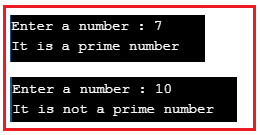##### Example: Fibonacci series

Let us create a Program to print the Fibonacci series up to a given number using a for loop in C Language.

```#include <stdio.h>
int main()
{
int n, n1 = 0, n2 = 1, temp;
printf("\nEnter a number : ");
scanf("%d", &n);
if(n >= 1)
{
printf("%d %d ", n1, n2);
temp = n1 + n2;

for(; temp <= n;)
{
printf("%d ", temp);
n1 = n2;
n2 = temp;
temp = n1+n2;
}
}
else
printf("please enter a number greater than zero");
}```
###### Output: